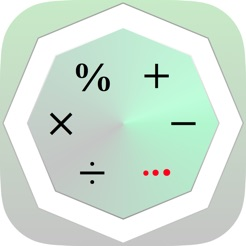Screenshots

•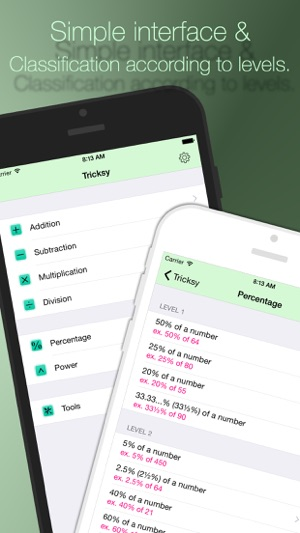•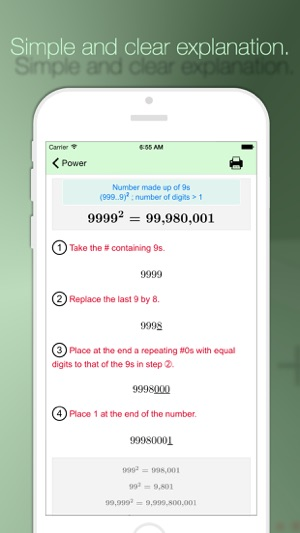•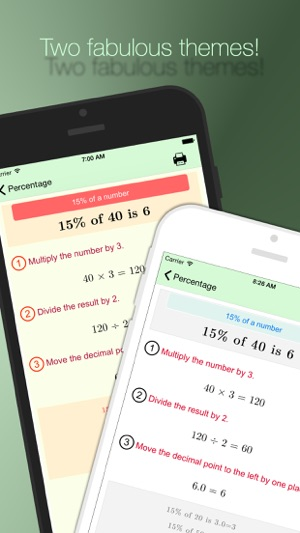•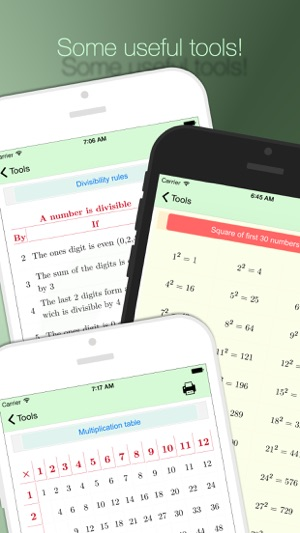•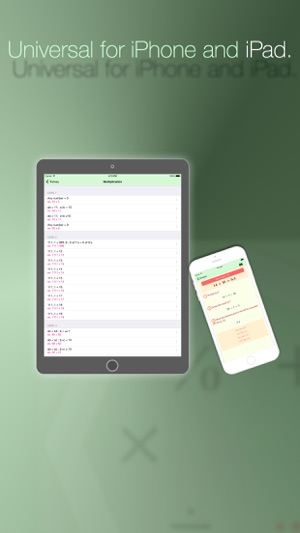Description

Tricksy is a universal app that allows you to solve many math operations in a simple way, by applying untraditional techniques.

This app includes more than 60 lessons classified into these domains: Addition, subtraction, multiplication, division, percentage and power.

These lessons are presented in levels, arranged from the easiest lesson to the most difficult one. Each of these lessons is explained in a simple and clear way; using steps supported by examples.

Besides the lessons, tricksy includes some useful tools for calculation, in which students can benefit, and may apply them during their school domain.

~The included tricks are:

• Adding two-digit numbers like ab + ba
• Adding 2 fractions like 1/a + 1/b
• Adding a sequence of odd numbers beginning by 1: 1 + 3 + 5 + … + 2n-1
• Adding a sequence of even numbers beginning by 2: 2 + 4 + 6 + … + 2n
• Adding a sequence from 1 to any number and back to 1 again: 1 + 2 + 3 +…+ X +…+ 3 + 2 +1
• Adding a sequence of doubles: X + 2⋅X + 2²⋅X + 2³⋅X + ... + 2ⁿ⋅X
• ...

▸ Subtraction:
• Subtracting two-digit numbers like ab − ba
• Subtracting 2 fractions like 1/a − 1/b
• Subtracting 2 fractions like c/a − c/b
• Subtracting 2 fractions like (a − 1)/a − (b − 1)/b
• Subtracting three-digit numbers like abc − cba
• Subtracting the squares of two numbers differing by 2: X² − (X−2)²
• ...

▸ Multiplication:
• Any number × 5
• Two digit number × 11: ab × 11; a + b < 10
• Two digit number × 11: ab × 11; a + b ≥ 10
• Any number × 9
• The repeated #1s × the repeated #9s having the same # of digits: 111..1 × 999..9
• The repeated #1s × 12: 111..1 × 12
• The repeated #1s × 13: 111..1 × 13
• The repeated #1s × 14: 111..1 × 14
• The repeated #1s × 15: 111..1 × 15
• The repeated #1s × 16: 111..1 × 16
• The repeated #1s × 17: 111..1 × 17
• The repeated #1s × 18: 111..1 × 18
• The repeated #1s × 19: 111..1 × 19
• Consecutive decade ending in 5: a5 × b5; b = a + 1
• Two 2-digit numbers with same 10s digit: ab × ac; b + c = 10
• Two 2-digit numbers with same 10s digit: ab × ac; b + c ≠ 10
• Two 2-digit numbers with same 1s digit: ab × cb; a + c = 10
• Two 2-digit numbers with same 1s digit: ab × cb; a + c ≠ 10

▸ Division:
• Any number ÷ 5
• Any number ÷ 50
• Any number ÷ 25
• Any number ÷ 250
• Any number ÷ 125
• Repeating 3-digit number ÷ 37: aaa ÷ 37
• Any number ÷ 40
• Any number ÷ 15
• Any number ÷ 35

▸ Percentage:
• 50% of a number
• 25% of a number
• 20% of a number
• 33.33...% (33⅓%) of a number
• 5% of a number
• 2.5% (2½%) of a number
• 40% of a number
• 60% of a number
• 70% of a number
• 75% of a number
• 45% of a number
• 15% of a number
• ...

▸ Power:
• Number made up of 1s: (111..1)² ; number of digits < 10
• Number made up of 3s: (333..3)²
• Number made up of 6s: (666..6)² ; 1 < number of digits
• Number made up of 9s: (999..9)² ; 1 < number of digits
• Two digit number beginning with 5: (5a)²
• Two digit number ending with 5: (a5)²

Challenge your brain to think efficiently while having fun with our smart math tricks !
--------------------------------------------

Version 1.4

• iOS 12 support

Ratings and Reviews

5.0 out of 5
5 Ratings

5 Ratings

amazing app

Lots of easy tricks to amaze your family and friends which are very useful in doing maths and are very easy to follow. Go for it! Thanks for this wonderful app :)

Ray2231 ,

Awesome app

Cool app something new and special

Love fisheye ,

Cool App

this app is interest for me ^^

Information

Seller
Hassan Taleb
Size
18.8 MB
Category
Education
Compatibility

Requires iOS 8.0 or later. Compatible with iPhone, iPad, and iPod touch.

Languages

English

Age Rating
Rated 4+
© 2017 Hassan Taleb
Price
\$2.99

Supports

•